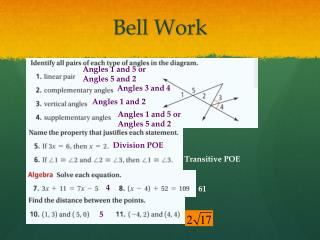DownloadDownload PresentationBell Work

# Bell Work

Download Presentation## Bell Work

- - - - - - - - - - - - - - - - - - - - - - - - - - - E N D - - - - - - - - - - - - - - - - - - - - - - - - - - -
##### Presentation Transcript

1. Bell Work Angles 1 and 5 or Angles 5 and 2 Angles 3 and 4 Angles 1 and 2 Angles 1 and 5 or Angles 5 and 2 Division POE Transitive POE 4 61 5

2. Parallel and Perpendicular Lines LEQ: What relationships exist between lines, planes and angles in space?

3. Parallel and Skew • Parallel Lines: Coplanar lines that do not intersect • Symbol: || • Example: plane ABCD || plane EFGH • Parallel Planes: planes that do not intersect • Skew Lines: noncoplanar and not parallel but still do not intersect

4. Angle Pairs formed by Transversal • Alternate Interior Angles: nonadjacent interior angles that lie on opposite sides of the transversal • Alternate Exterior Angles: nonadjacent exterior angles that lie on opposite sides of the transversal 1 2 4 3 6 5 8 7

5. Same side interior angles • Interior angles that lie on the same side of the transversal • Angles 4 and 5 or Angles 3 and 6 1 2 4 3 6 5 8 7

6. Corresponding Angles • Lie on the same side of the transversal and in corresponding positions • Same spot on different line • Angles 1 and 5 • Angles 4 and 8 • Angles 2 and 6 • Angles 3 and 7 1 2 4 3 6 5 8 7

7. IDENTIFY EF CD • Line parallel to AB • Line parallel to BF • Plane parallel to plane ABC • Plane parallel to plane EFB • A line that is skew to AD • A line that is skew to EF CG AE D C B A Plane EFG Plane GCD G BF CG EF BC E F

8. Corresponding Angles Postulate • If a transversal intersects two parallel lines, then corresponding angles are congruent r 1 2 4 3 m 5 6 8 7 p

9. Alternate Interior Angles Theorem • If a transversal intersects two parallel lines, then alternate interior angles are congruent. r 4 3 m 5 6 p

10. Same-side Interior Angles Theorem • If a transversal intersects two parallel lines, then same-side interior angles are supplementary r 4 3 m 5 6 p

11. Alternate Exterior Angles Theorem • If a transversal intersects two parallel lines, then alternate exterior angles are congruent. r 1 2 m 8 7 p

12. Finding Congruent Angles • Find all the Angles that are congruent to the given value and justify your answer: 1 2 4 5 = 120° (Vertical) 3 = 120° (Corresponding) 1 = 120° (Alt. Exterior) 3 5 6 8 120°

13. Finding Angle Measures 3 = 120° (Corresponding) • Find angle 3 and angle 2: 1 2 4 3 2 + 120° = 180° (SS Ext.) 2 = 60° 5 6 8 120°

14. Solve for x x + (x – 26) = 180 x = 103 2x – 26 = 180 2x = 206